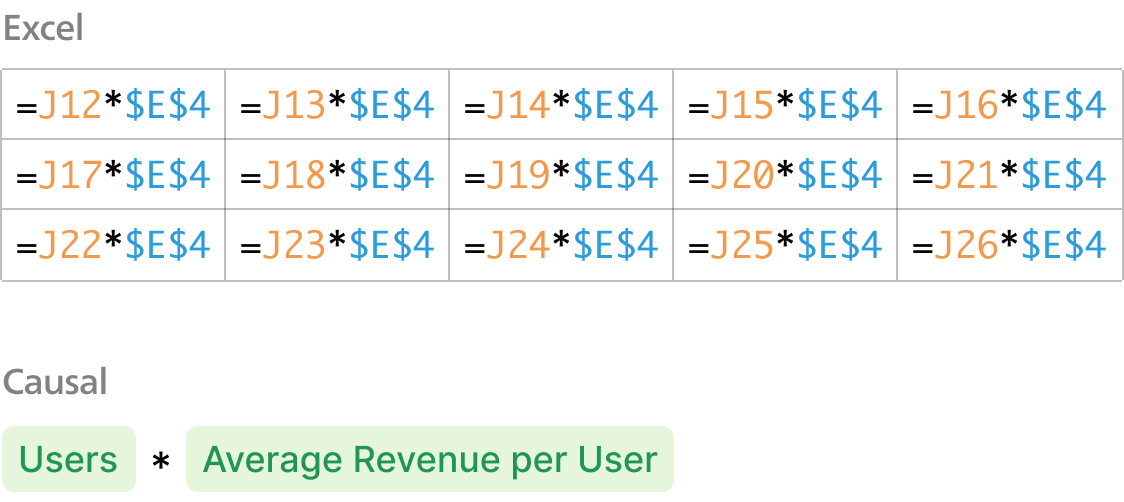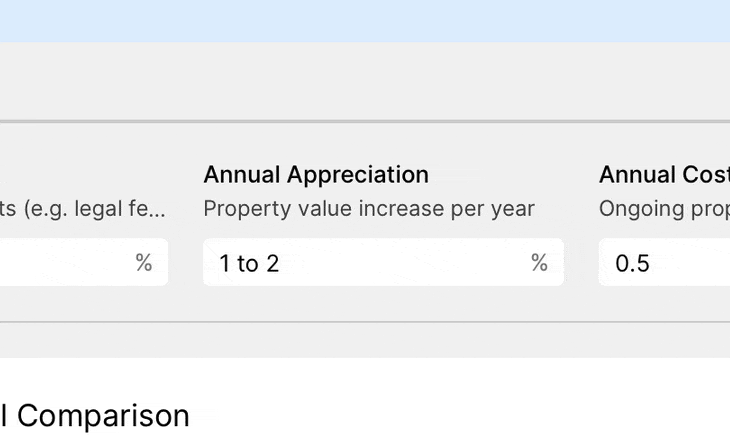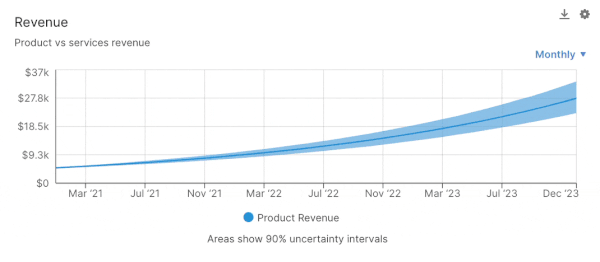# How to Calculate Debt to Equity Ratio in Sage Intacct

## What is Debt to Equity Ratio?

Debt to Equity Ratio is a financial ratio that measures a company's financial leverage. It is calculated by dividing total liabilities by total shareholder equity.

The higher the ratio, the more debt the company has.

## How do you calculate Debt to Equity Ratio in Sage Intacct?

It can be difficult to calculate Debt to Equity Ratio directly inside of Sage Intacct; that's where Causal comes in.

Causal is a modelling tool which lets you build models on top of your Sage Intacct data. You simply connect Causal to your Sage Intacct account, and then you can build formulae in Causal to calculate your Debt to Equity Ratio.

## What is Causal?

Causal lets you build models effortlessly and share them with interactive, visual dashboards that everyone will understand.

In Causal, you build your models out of variables, which you can then link together in simple plain-English formulae to calculate metrics like Debt to Equity Ratio. This makes your models easy to understand and quick to build, so you can spend minutes, not days, on your models.When you're done, you can share the link to your model with stakeholders. They'll be able to view your model's outputs in a visual dashboard, rather than a jumble of tabs and complex formulae. The dashboards are interactive, letting viewers tweak your assumptions to see how they affect the model's outputs.Causal lets you add visuals in a single click, letting you plot out graphs and distributions for metrics like Debt to Equity Ratio.#### data

Thanks! We'll be in touch soon.
Oops! Something went wrong while submitting the form.